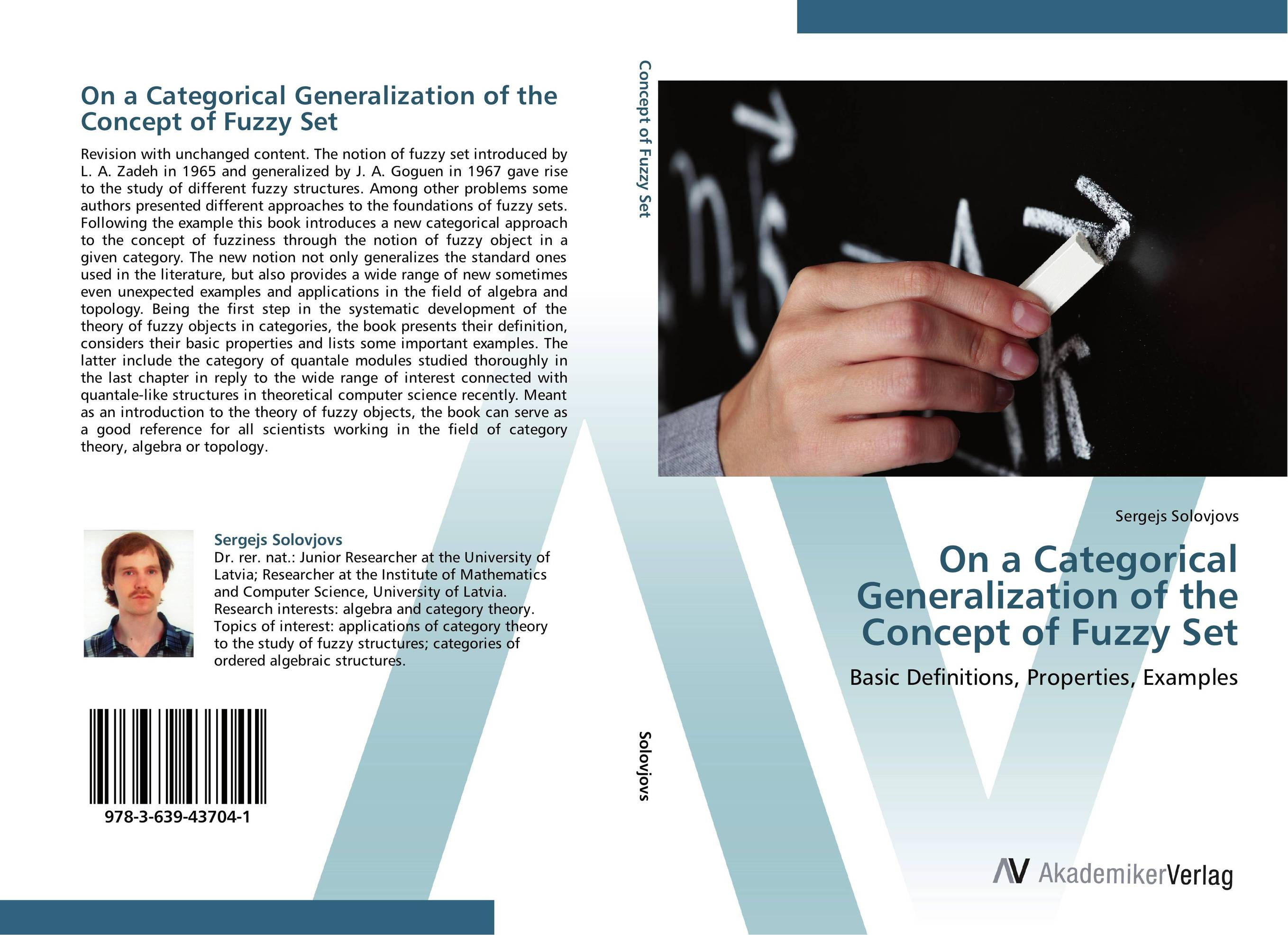# On a Categorical Generalization of the Concept of Fuzzy Set

###### Аннотация:
Revision with unchanged content. The notion of fuzzy set introduced by L. A. Zadeh in 1965 and generalized by J. A. Goguen in 1967 gave rise to the study of different fuzzy structures. Among other problems some authors presented different approaches to the foundations of fuzzy sets. Following the example this book introduces a new categorical approach to the concept of fuzziness through the notion of fuzzy object in a given category. The new notion not only generalizes the standard ones used in the literature, but also provides a wide range of new sometimes even unexpected examples and applications in the field of algebra and topology. Being the first step in the systematic development of the theory of fuzzy objects in categories, the book presents their definition, considers their basic properties and lists some important examples. The latter include the category of quantale modules studied thoroughly in the last chapter in reply to the wide range of interest connected with quantale-like structures in theoretical computer science recently. Meant as an introduction to the theory of fuzzy objects, the book can serve as a good reference for all scientists working in the field of category theory, algebra or topology.
###### Издания произведения:9783639437041

### Рекомендуем

#### Комментарии

Пока нет ни одного комментария
Вы должны войти для того что бы оставлять комментарии